## Chapter 11. Configuration Interaction

Abstract

AMPAC™ offers users a vastly improved and expanded configuration interaction (CI) capability when compared to other semiempirical programs. Given the special keywords and preselection techniques that have been implemented, AMPAC can now perform both extremely complex, yet efficient CI calculations.

Introduction
CI Theory
Total Electron Density and Electron Spin Density Properties
Transition Dipole Moments and Oscillator Strengths
Dynamic Polarizabilities
The CI Active Space
Open-Shell RHF: The Half-Electron Method
The Initial CI Microstate Space
The Truncated CI Microstate Space
CI Input
Explicitly Invoking CI
Implicitly Invoking CI
Active Space Definition
Microstate Selection
Primary CI Eigenstate Selection
CI Output Control
CI-Specific Properties
Job Control
Extra Input Data
Other Keywords of Interest
Keywords Incompatible With CI
Configuration Interaction Dedicated Keywords

## Introduction

The general-purpose semiempirical models available in AMPAC were originally developed for the efficient prediction of ground state molecular properties at equilibrium geometries. This is where the models were parameterized and where the vast majority of data exists. The level of theory upon which these models are based is the Hartree-Fock SCF method, restricted or unrestricted, which has proved sufficiently accurate to reproduce and predict ground-state properties of most ordinary organic molecules and many molecules containing only main-group elements. For excited state geometries and properties, some open-shell systems, atoms or molecules containing metals (e.g., transition metal complexes), properties which involve excited states (e.g., UV/visible spectra) and unusual geometries (e.g., transition states corresponding to weakly avoided crossings) the Hartree-Fock level of theory is often inadequate at best. The need for efficient semiempirical methods capable of handling these cases has long been recognized, especially for larger molecules. Dedicated methods parameterized specifically for some of these cases (e.g., UV/visible spectra) have been proposed and implemented by others. In AMPAC, the approach taken is to use a post-SCF configuration interaction (CI) method, starting with a semi-empirical SCF wavefunction already available. In semiempirical models, some of the non-specific electron correlation is absorbed by the parameterization and a crude large-scale CI is usually meaningless. Therefore, an adapted CI capable of modeling primarily the specific electron correlation necessary to describe excited states and open-shells systems is usually preferable.

## CI Theory

An AMPAC N-electron wavefunction Ψ is always initially defined to be a single-determinant SCF wavefunction ΨSCF, i.e., a determinant ψ of orthonormal, variationally determined spin orbitals (SOs) χ. For RHF, the spatial components (MOs) φ of the SOs are restricted to be identical in pairs of alpha and beta electrons (or half-electrons of fractional charge for open-shell RHF) during the SCF, whereas this is not the case in UHF. In some RHF cases, it is desirable or necessary (e.g., open-shell RHF, calculation of UV/visible spectra, etc.) to go beyond SCF to a more sophisticated Configuration Interaction (CI) method (CI cannot be used with UHF), where ψ = ψCI is one of many possible variationally determined linear combinations of determinants ψ. The set of determinants ψ combined to form ΨCI is called an N-electron basis (in contrast, to the one-electron basis of Slater orbitals used to expand the SOs) and includes a reference determinant ΨRef (usually ΨSCF) as well as a set of excited determinants obtained by moving one or more of the electrons from the occupied SOs of ΨRef to corresponding virtual SOs. For open-shell RHF, the reference wavefunction ΨRef is not ΨSCF since it contains fractionally occupied SOs, but rather a determinant obtained by filling the SOs of ΨSCF in the standard way using the Aufbau principle. In AMPAC, a generic single determinant ψ is referred to as a microstate. A general expression for ΨCI can be given by:(11.1)

where the Cs are the linear coefficients to be determined, ψia is the determinant resulting from moving an electron from occupied SO χi to virtual SO χa, ψia,jb is the determinant resulting from moving electrons from occupied SOs χi and χj to virtual SOs χa and χb respectively, etc. The sums involving i, j, k, ... are over some subset of occupied SOs in ψRef while the sums involving a,b,c,... are over some subset of virtual SOs in ψRef. Together, these SOs define the CI-active MOs, or the active space. A set of CI wavefunctions and corresponding energies can be variationally determined (coefficients C optimized, orbitals fixed) by solving the matrix eigenvalue equation resulting from differentiating the standard Hamiltonian energy expression with respect to the elements of the CI coefficient vector C and setting the result to zero: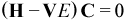(11.2)

where H is a semi-empirical Hamiltonian matrix over microstates (Hpq = <ψp|Hq >) and V is the overlap matrix over microstates (Vpq = <ψpq>).

Members of the set of CI wavefunctions satisfying Equation (11.2) are called CI eigenstates and are labeled here by their root number in order of increasing energy as Ψ[R], starting with R = 1 for the lowest energy CI eigenstate Ψ.

A microstate ψ with Nα alpha electrons and Nβ beta electrons is an eigenfunction of the operator Ŝz (the z-component of the total electron spin angular momentum operator) with eigenvalue Sz:(11.3)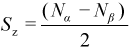(11.4)

For an N-electron system, the set {Sz} of possible values for Sz is:(11.5)

A microstate ψ is not an eigenfunction of the operator Ŝ2 (the square of the total electron spin angular momentum operator) unless it has a closed-shell configuration (all MOs doubly occupied or empty) or a high-spin open-shell configuration (all singly-occupied MOs have parallel spin):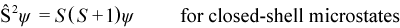(11.6)

A spin adapted microstate η[S,Sz] is a linear combination of microstates that is defined to be an eigenfunction of both Ŝz and Ŝ2:(11.7)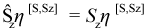(11.8)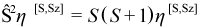(11.9)

For example, a microstate with two singly-occupied MOs with opposite spin (Sz = 0) is not an eigenfunction of Ŝ2, but the combination of this microstate with the corresponding spin-flipped microstate is an eigenfunction of Ŝ2, with quantum number S = 0.

For an N-electron system, the set of possible values for S are:(11.10)

For a given value of S, the set of possible values of Sz are: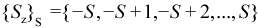(11.11)

The spin multiplicity SM corresponding to S is given by: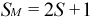(11.12)

The spin multiplicity indicates the number of possible values for Sz, and therefore the degeneracy of a spin adapted microstate with total spin quantum number S. For singlets (SM = 1, S = 0), there is only one possibly value for Sz: Sz = 0. For doublets (SM = 2, S = 1/2), there are two possible values for Sz: Sz = -1/2 and Sz = 1/2. For triplets (SM = 3, S = 1), there are three possible values for Sz: Sz = -1, Sz = 0 and Sz = 1.

Since exact eigenstates of the non-relativistic Hamiltonian (modeled by the semi-empirical Hamiltonian H) are pure spin states, i.e., eigenfunctions of both Ŝz and Ŝ2, it useful to constrain the CI eigenstates to be as well. This can be achieved if, instead of using just the raw microstates ψ as the N-electron basis prior to solving Equation (11.2), the N-electron basis is defined in terms of spin adapted microstates η.

In terms of a set of spin adapted microstates η[S,Sz] with spin quantum numbers S and Sz, a corresponding pure spin state CI eigenstate Ψ[R,S,Sz]CI is given by: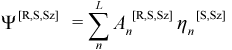(11.13)

In AMPAC, the CI eigenstates generated are always expanded according to Equation (11.13) and so they are pure spin states. In addition, for efficiency only one member of a degenerate set of spin adapted microstates is ever used. By default, this is the one with the smallest non-negative value of Sz (0 for even-electron systems, 1/2 for odd-electron systems), but this is modifiable using the keywords SZ=`n` or MICROS=`n`. Thus, the CI matrix equations to be solved are: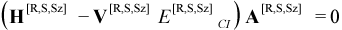(11.14)

where H[R,S,Sz]pq = <ηp[S,Sz]|H|ηq[S,Sz]> and Vpq = <ηp[S,Sz]q[S,Sz]>.

Combining equations Equation (11.7) and Equation (11.13), Ψ[R,S,Sz]CI can be expressed directly in terms of the microstates ψm[Sz] with coefficients Dm[R,S,Sz] as: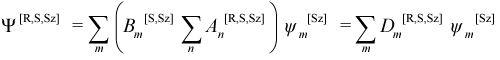(11.15)

In the AMPAC output files, it is always the microstate coefficients Dm[R,S,Sz] which are printed.

While there are many CI eigenstates which can be calculated (the number can be specified using the keyword CISTATE=`n`), AMPAC considers one of them to be the primary CI eigenstate whose energy hypersurface will be followed during geometry optimizations and which will be used as the reference for all property calculations. The other n - 1 CI eigenstates requested by CISTATE=`n` are considered secondary CI eigenstates, for which some properties are calculated and printed, typically at one or more optimized geometries of the primary eigenstate, so their transition properties are non-adiabiatic. By default, the primary CI eigenstate is the ground state, of any spin multiplicity, and the secondary eigenstates are all excited states. To specify a different primary CI eigenstate, use one of the spin multiplicity keywords SINGLET, DOUBLET, TRIPLET, etc. and/or ROOT=`n`, where n = 1 refers to the ground state. For example, to use the second‑lowest energy triplet CI eigenstate (T2) as the primary one, specify TRIPLET and ROOT=2. To use the second‑lowest energy CI eigenstate of any spin multiplicity as the primary one, specify ROOT=2 without a spin multiplicity keyword.

### Total Electron Density and Electron Spin Density Properties

For the CI eigenstate Ψ[R,S,Sz], the total electron density function ρ[R,S,Sz](r) is expressed in terms of the 2M occupied and virtual SOs χ of ψRef by:(11.16)

where γmi is the occupancy (0 or 1) of the ith SO for the mth microstate, sz,i = 1/2 for alpha SOs and -1/2 for beta SOs. In terms of the corresponding M MOs, ρ[R,S,Sz](r) is given by:(11.17)

where γa,mi is the occupancy (0 or 1) of the alpha SO of the ith MO for the mth microstate and PMO[R,S,Sz] is the total one-electron density matrix in the MO basis, with alpha and beta contributions PMO,α[R,S,Sz] and PMO,β[R,S,Sz], respectively. In terms of a basis of L atomic orbitals (AOs) symbolized by ξ, ρ[R,S,Sz](r) is given by:(11.18)

where PAO[R,S,Sz] is the total one-electron density matrix in the AO basis, with alpha and beta contributions PAO,α[R,S,Sz] and PAO,β[R,S,Sz], respectively.

In AMPAC, when the keyword CIDIP is specified, the dipole moment and Mulliken atomic charges are calculated for both the primary and secondary CI eigenstates from the corresponding density matrices PAO[R,S,Sz]. In general, other one-electron properties which are also available without CI, such as ESP charges, are calculated in CI calculations from PAO[R,S,Sz], but only for the primary CI eigenstate.

The electron spin density ρσ[R,S,Sz](r) corresponding to ρ[R,S,Sz](r) is simply the alpha electron density ρα[R,S,Sz](r) minus the beta electron density ρβ[R,S,Sz](r), which, along with the corresponding spin density matrices PMO,α[R,S,Sz] and PAO,β[R,S,Sz] is given by: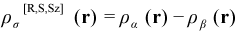(11.19)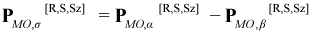(11.20)(11.21)

In AMPAC, when the ESR keyword is specified, the spin density matrices PMO,α[R,S,Sz] and PAO,β[R,S,Sz] are printed for the primary CI eigenstate along with the net Mulliken atomic electron spins for both primary and secondary CI eigenstates. The net Mulliken electron spin for the Ath atom, σA, is calculated like the corresponding Mulliken atomic electron population except that PAO,σ[R,S,Sz] is used instead of PAO[R,S,Sz]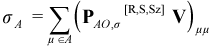(11.22)

### Transition Dipole Moments and Oscillator Strengths

The transition dipole moment μ[R→n,S,Sz] between CI eigenstates Ψ[R,S,Sz] and Ψ[n,S,Sz] is an important result: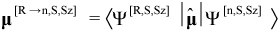(11.23)

For example, contributions from all available transition dipole moments appear in the sum-over-states (SOS) expression for the dynamic polarizability tensor α[R→n,S,Sz](ω), given by Equation (11.26). Individual transition dipole moments are also of interest because they yield information about the UV / visible spectrum of a molecule. The oscillator strength f[R→n,S,Sz]between states ψ[R,S,Sz] and ψ[n,S,Sz] is proportional to the absorptivity of light at a wavelength λ[R→n,S,Sz]: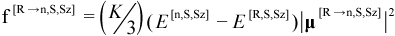(11.24)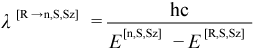(11.25)

where K is a constant. By default, AMPAC writes the transition dipole moments μ[R→n,S,Sz], transition wavelengths λ[R→n,S,Sz] and oscillator strengths f[R→n,S,Sz] between the primary eigenstate Ψ[R,S,Sz] and all of the secondary CI eigenstates Ψ[n,S,Sz]. In AMPAC, the number of CI eigenstates to calculate, including the primary CI eigenstate, can be specified using the CISTATE=`n` keyword (some of these will have a different total spin quantum number S than the primary eigenstate and so their corresponding transition dipole moments vanish).

### Dynamic Polarizabilities

The sum-over-states (SOS) expression for the dynamic polarizability tensor α[R→n,S,Sz](ω) for the CI eigenstate ψ[R,S,Sz] is given by:(11.26)

where ω is the external electric field frequency (in energy units) and the sum is over all possible CI eigenstates different from the primary eigenstate, but having the same S and Sz quantum numbers. In AMPAC, α[R→n,S,Sz](ω) will be calculated and written to the AMPAC output file when the keywords DYNPOL or DYNPOL=`n.nnnn` are specified. Note that the keyword CISTATE=`n` has no influence on the calculation of dynamic polarizabilities, and vice versa, but the number of possible CI eigenstates (determined by the active space and hence the number of final microstates) does.

### The CI Active Space

The set of occupied and virtual MOs whose corresponding SOs are allowed to exchange electrons in ΨRef to form new microstates ψ are called the CI-active MOs or the active space. The choice of active space is one of the most crucial, and sometimes difficult, steps in a CI calculation, both computationally and in terms of physical results. Given this importance, the CI-active MOs are usually specified along with the keywords which invoke CI, possibly together with the RECLAS(`n,m`) keyword and its associated MO permutation data. For example, C.I.(5,8) means do a CAS-CI using MOs 5,6,7 and 8 as the CI-active MOs. It is essential that all or none of the members of a degenerate set of MOs be included in the active space. By default, AMPAC will abort if this is not the case. The keywords, CIGAP=`n.nnnn` and CI-OK can be used to alter the definition of MO degeneracy and to allow the active space to contain an incomplete set of degenerate MOs. By default, all of the MO energies are printed to the AMPAC output file. The keywords VECTORS and ALLVEC can be used to print both the MO energies and AO coefficients to the AMPAC output file for inspection. This information is also present in an AMPAC visualization file so that MOs can be visualized with AMPAC’s GUI. It is important to know the order in which the SCF MOs occur and their corresponding labeling. For a system with M MOs, the MOs are ordered from 1 to M by increasing occupancy, i.e., first doubly-occupied MOs, then partially occupied MOs and finally unoccupied (virtual) MOs. This order usually coincides with increasing MO energy for the entire list from 1 to M, but within each subset of the same occupancy the order always coincides with increasing energy.

### Open-Shell RHF: The “Half-Electron” Method

For RHF open-shell calculations, the SCF calculations in AMPAC are done using the Half-Electron method instead of the ROHF (Restricted Open-Shell Hartree-Fock) method used by others. In the Half-Electron method, the usual spin-less closed-shell RHF SCF formalism is used to calculate ΨSCF, except that instead of N / 2 doubly occupied spatial MOs there are assumed to be N / 2 - n (N even) or N / 2 + 1 - n (N odd) doubly occupied MOs and m MOs with fractional occupancies which sum to n, where n is the number of open-shell electrons . When the OPEN(`n,m`) keyword is specified, the m open MOs have an equal occupancy of (n/m). When the SCFCI(`n,m1,m2,r`) keyword is specified, the set of m = m1 + m2 open MOs consists of a group of m1 MOs each with an occupancy of (nm1)/(m1+rm2) and a group of m2 MOs each with an occupancy of (nrm2)/(m1+rm2). A fractionally occupied MO in the Half-Electron method may be thought of as being occupied by two half-electrons of opposite spin and with a charge equal to half the occupancy of the MO, e.g., (n / 2m) when OPEN(`n,m`) is used. This leads to an energy expression which is similar to Roothan’s multiconfiguration open-shell SCF energy expression after spurious coulomb and exchange energies arising from the interaction between half-electrons are subtracted out. In AMPAC, however, the energy calculated using the Half-Electron method is never used, since it is non-variational, but the corresponding set of SCF MOs are, either in a minimal CAS-CI calculation involving all of the partially occupied MOs of ΨSCF as the active space if CI is not otherwise invoked, or more generally in any specified type of CI calculation. While the fractionally occupied SOs of ΨSCF determine the active space of corresponding MOs, the reference wavefunction ΨRef used from an open-shell RHF calculation is not ΨSCF but rather a determinant obtained by filling the SOs of ΨSCF in the standard way using the Aufbau principle. It is important to note that, in general, the number of open-shell electrons to assume for the SCF should be specified explicitly using one of the keywords OPEN(`n,m`), BIRADICAL, EXCITED or SCFCI, otherwise AMPAC will assume the minimum number of open-shell electrons (0 for even-electron systems and 1 for odd-electron systems) for the SCF. The spin-multiplicity keywords (e.g., SINGLET, DOUBLET, TRIPLET, etc.) are not used in RHF until the CI portion of the calculation. Thus, for the oxygen molecule, OPEN(2,2) should be specified even if TRIPLET is also specified.

### The Initial CI Microstate Space

Given ψRef and a corresponding active space, a definition of which microstates to generate and potentially use for the expansion of the CI eigenstates is necessary. In the Complete Active Space method (CAS-CI), specified by C.I.=`n` or C.I.(`n,m`) and the default when CI is only implied by OPEN(`n,m`), all possible microstates which can be generated by permutations of the electrons among the SOs within the active space are potentially used. In the CI Singles method (S-CI), specified by SC.I.=`n` or SC.I.(`n.m`), all possible singly-excited microstates ψia are potentially used. In the CI Singles and Doubles method (SD-CI), specified by SDC.I.=`n` or SDC.I.(`n,m`), all possible singly-excited microstates ψia and doubly-excited microstates ψia,jb are potentially used. In the CI Singles, Doubles and Triples method (SDT-CI), specified by SDTC.I.=`n` or SDTC.I.(`n,m`), all possible singly-excited microstates ψia, doubly-excited microstates ψia,jb and triply-excited microstates ψia,jb,kc are potentially used. The initial set of microstates is referred to here as {I}MS.

### The Truncated CI Microstate Space

The size of {I}MS grows very rapidly (combinatorially) as the size of the active space increases, especially when CAS-CI is used. (For a CAS-CI involving 10 electrons and 10 CI-active MOs, the number of possible microstates is over 60000, after spin degeneracies are excluded.) In some cases, all of {I}MS should be used, if possible. If this is not the case, whether due to resource limitations and / or to avoid over-correlating the already partially correlated, semi-empirically calculated ground state energy, then some means of efficiently selecting the most important final set of microstates, referred to here as {F}MS, from {I}MS is necessary. Typically, only a relatively small target set of the possible CI eigenstates, {R}ES, are of interest. For example, {R}ES might be composed of the singlet ground CI eigenstate and the first excited singlet and triplet CI eigenstates. {R}ES can usually be characterized in terms of relatively large contributions from a small subset of germ microstates {G}MS = {G0, G1, G2, ?}MS, where G 0≡ ψRef roughly corresponds to the ground CI eigenstate R0, G1 to a first excited CI eigenstate R1, etc. While, much of the information relevant to {R}ES is included in {G}MS, the CI eigenstates of {R}ES constructed from {G}MS alone would generally have two significant deficiencies. First, there is generally a lack of specific correlation within the set {G}MS. Second, the excited members {G}MS are lacking in repolarization because the SCF orbitals from which {G}MS is generated are obtained from a ground state wavefunction optimization. The objective of the microstate selection procedure used in AMPAC to produce {F}MS is to extract from the enormous list of initial microstates in {I}MS and not in {G}MS, the ones which should contribute most to specific correlation and repolarization. This microstate selection consists of four major steps:

1. From the initial microstate space {I}MS, keep those J1(≈ 10 × J4, J4 defined below) microstates ψ with the lowest Møller‑Plesset zero‑order energy E0MP[ψ] (sum of occupied SO energies):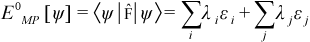(11.27)

where the sums over i and j are over all alpha and beta SOs, respectively, while λ and ε represent SO occupancies and energies, respectively.

2. From the J1 microstates of step I, choose the J2 (default 100) microstates ψ with the lowest Epstein‑Nesbet (EN) energy EEN[ψ] (semi‑empirical Hamiltonian expectation value).(11.28)

This set of J2 microstates is the germ set {G}MS referred to above.

3. From {G}MS of step II, determine the J3 (default 30) eigenvectors of the corresponding CI matrix.

4. From the J1 - J2 non-germ microstates ψ which are in {I}MS but not {G}MS, choose the J4 (default 1200) - J2 microstates which make the largest contribution to the following quantity W[ψ]:(11.29)

At each stage of this microstate selection procedure, the sets of microstates selected are required to preserve spatial degeneracy, i.e., all members of a degenerate set of microstates are kept if there is space available in the target list, or not kept if there is not space available in the target list. This is achieved by simple inspection of the Møller-Plesset zero-order energies, using a degeneracy threshold of 1.0 × 10-4 eV, which is adjustable by the keyword CIGAP=`n,n`. Of course, this procedure will not cover the case of an active space containing only a partial set of degenerate MOs. It is important to remember that either all or none of the members of a degenerate set of MOs should be included in the active space.

In AMPAC, the above microstate selection procedure can be partially customized by specifying the parameters J2, J3 and J4 using the keywords CIMAX=J4, PERTU=J2 and PERTU(J2,J3).

## CI Input

### Explicitly Invoking CI

The following keywords will cause a CI calculation to be done following an RHF calculation:

### Implicitly Invoking CI

The following keywords will cause at least a minimum CAS-CI calculation to be done following an RHF calculation. These may also be combined with a keyword which explicitly invokes a larger CI calculation:

NoteAn odd electron system without UHF specified and without an explicit C.I. keyword will implicitly invoke OPEN(1,1), which in turn invokes a minimum CAS-CI calculation.

### Active Space Definition

The CI-active MOs are always at least least initially defined by the keywords which invoke CI, possibly together with the RECLAS(n,m) keyword. The following keywords modify this definition or have an important bearing on it:

### Microstate Selection

Given an initial set of microstates {I}MS defined by the CI-active MOs, the following keywords help determine the number and nature of the final microstates {F}MS actually used in the CI eigenstate expansions:

### Primary CI Eigenstate Selection

The following keywords determine the spin multiplicity SM, CI root and handling of degeneracies (EPS) for the primary CI eigenstate, as well as the number and kind of secondary CI eigenstates calculated:

### CI Output Control

The following keywords control what kind of extra information is printed to the AMPAC output file or other result files (CIOUT) following any CI calculation:

### CI-Specific Properties

The following keywords can be used to calculate some extra molecular properties which are specific to a CI calculation:

### Keywords Incompatible With CI

Specifying any of the following keywords together with a keyword which either explicitly or implicitly invokes CI is an error, and will result in AMPAC aborting the job.

 Not allowed due to the absence of molecular orbitals. CI calculations cannot be reliably restarted. CI requires RHF. Not available for methods using analytical CI gradients. CI not implemented with sparse matrices. CI requires RHF.

## Configuration Interaction Dedicated Keywords

 Averaged density matrix in MO basis for the first `n` eigenstates. System has two unpaired electrons. Include n orbitals around the HOMO in the CI manifold. Override degeneracy check. Calculate charges and dipole moments for CI eigenstates. Specify energy gap used to determine microstate degeneracy. Specify the maximum number of microstates. Write details about the CI eigenstates to file. Specify the number of final CI eigenstates to be calculated and printed. Calculate and write transition dipole data between all calculated CI eigenstates instead of just between the primary CI eigenstate and the others. Write details about the CI matrix diagonalization to file. RHF decet state required. RHF doublet state required. Outputs data for dynamic polarizability calculations. Unpaired spin density on atoms will be calculated. First excited singlet state will be optimized. Require use of defined set of prototype MOs. Read final microstates from an ASCII file. All unique two electron integrals over CI-active MOs written to output file. Energies and AO coefficients of CI-active MOs printed to output file. Print information about CI microstates and transitions. Generates only microstates with spin = `n`. Maximum charge for generated microstates Constrains the spin multiplicity of the primary CI eigenstate to be n. RHF nonet state required. Expand space of single excitations in a CI calculation. RHF octet state required. Configuration Interaction. Define prototype MOs. Override the default perturbative selection of microstates. RHF quartet state required. RHF quintet state required. Reorder MOs. Propagate initial selection of microstates throughout a geometry optimization. Specify spin state to follow. Defines two sets of open-shell MOs and their fractional occupancies to be used in a “half-electron” RHF SCF calculation preceding a CI calculation. Specify CI-active MOs in a S-CI calculation. Specify CI-active MOs in a SD-CI calculation. Specify CI-active MOs in a SDT-CI calculation. Specify energy gap used to determine eigenstate degeneracy. RHF singlet state required. RHF septet state required. RHF sextet state required. Specify value of Sz. Triplet state required. Indicate that the microstates to be read in are fully consistent.